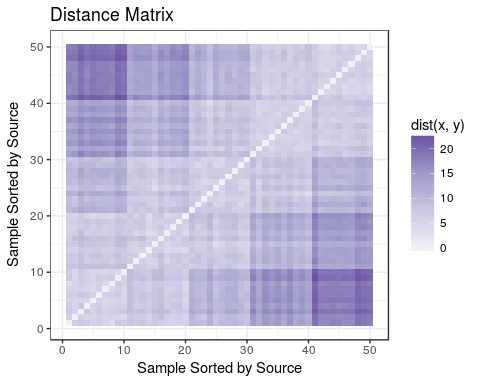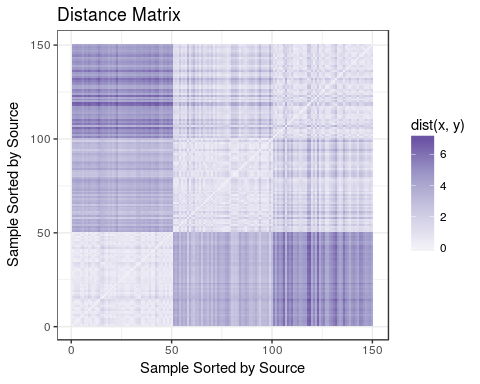# Discriminability Statistic

In this notebook, we will discuss the basics of the discriminability statistic in a real dat simulated and real data context, and demonstrate some of the useful plots that users may want to visualize the results with.

library(mgc)
library(reshape2)
library(ggplot2)

plot_mtx <- function(Dx, main.title="Distance Matrix", xlab.title="Sample Sorted by Source", ylab.title="Sample Sorted by Source") {
data <- melt(Dx)
ggplot(data, aes(x=Var1, y=Var2, fill=value)) +
geom_tile() +
colours=c("#f2f0f7", "#cbc9e2", "#9e9ac8", "#6a51a3"),
limits=c(min(Dx), max(Dx))) +
xlab(xlab.title) +
ylab(ylab.title) +
theme_bw() +
ggtitle(main.title)
}

## Simulated Data

Here, we assume that we have 5 independent sources of a measurement, and take 10 measurements from each source. Each measurement source i has measurements sampled from N(i, I_d) where d=20.

nsrc <- 5
nobs <- 10
d <- 20
set.seed(12345)
src_id <- array(1:nsrc)
labs <- sample(rep(src_id, nobs))
dat <- t(sapply(labs, function(lab) rnorm(d, mean=lab, sd=1)))
discr.stat(dat, labs)  # expect high discriminability since measurements taken at a source have the same mean and sd of only 1
##  0.9224444

we may find it useful to view the distance matrix, ordered by source label, to show that objects from the same source have a lower distance than objects from a different source:

Dx <- as.matrix(dist(dat[sort(labs, index=TRUE)$ix,]), method='euclidian') plot_mtx(Dx)as we can see, the ordering of the data elements does not matter, and users can pass in the data as either an $$[n, d]$$ array, or a $$[n, n]$$ distance matrix: discr.stat(Dx, sort(labs)) ##  0.9224444 ## Real Data Below, we show how discriminability might be used on real data, by demonstrating its usage on the first $$4$$ dimensions of the iris dataset, to determine the relationship between the flower species and the distances between the different dimensions of the iris dataset (sepal width/length and petal width/length): Dx <- as.matrix(dist(iris[sort(as.vector(iris$Species), index=TRUE)$ix,c(1,2,3,4)])) plot_mtx(Dx)we expect a high discriminability since the within-species relationship is clearly strong (the distances are low for same-species): discr.stat(iris[,c(1,2,3,4)], as.vector(iris$Species))
##  0.9320476

which is reflected in the high discriminability score.